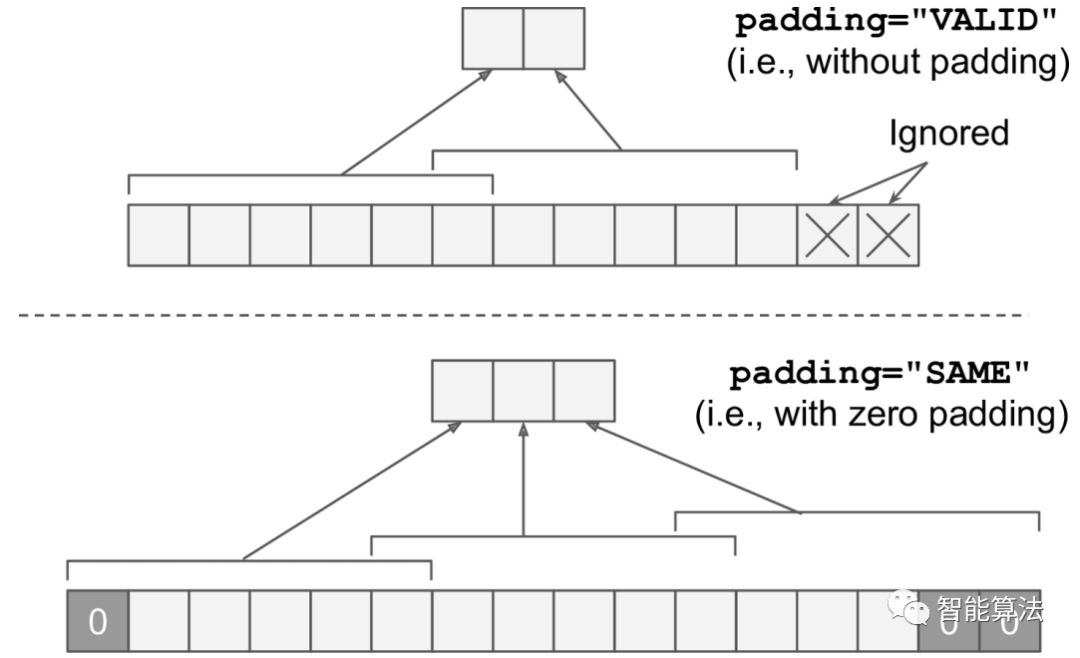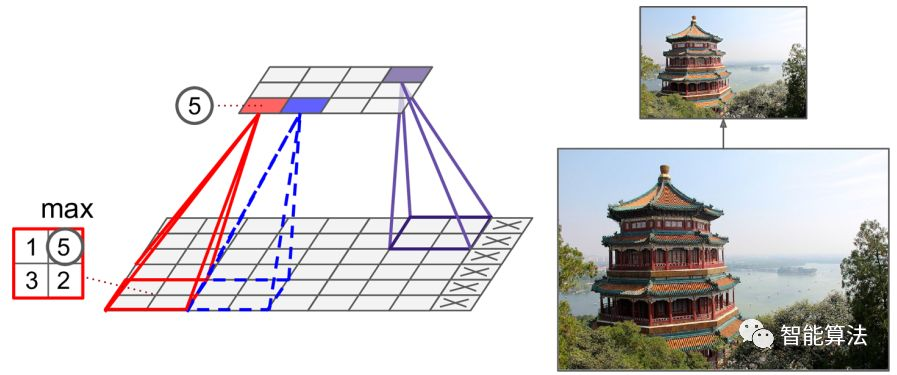# 深度学习三人行(第9期)----卷积神经网络实战进阶(附代码)

2018/11/22 08:00

• CNN实现(TensorFlow)

• CNN之内存计算

• CNN之池化层

• 小结

 1import numpy as np 2from sklearn.datasets import load_sample_images 3# Load sample images 4dataset = np.array(load_sample_images().images, dtype=np.float32) 5batch_size, height, width, channels = dataset.shape 6# Create 2 filters 7filters_test = np.zeros(shape=(7, 7, channels, 2), dtype=np.float32) 8filters_test[:, 3, :, 0] = 1 # vertical line 9filters_test[3, :, :, 1] = 1 # horizontal line10# Create a graph with input X plus a convolutional layer applying the 2 filters11X = tf.placeholder(tf.float32, shape=(None, height, width, channels))12convolution = tf.nn.conv2d(X, filters, strides=[1,2,2,1], padding="SAME")13with tf.Session() as sess:14    output = sess.run(convolution, feed_dict={X: dataset})15plt.imshow(output[0, :, :, 1]) # plot 1st image's 2nd feature map16plt.show()(5x5x3+1)x200 = 15200

200x150x100x32 = 96million bits1[...] # load the image dataset, just like above2# Create a graph with input X plus a max pooling layer3X = tf.placeholder(tf.float32, shape=(None, height, width, channels))4max_pool = tf.nn.max_pool(X, ksize=[1,2,2,1], strides=[1,2,2,1],padding="VALID")5with tf.Session() as sess:6    output = sess.run(max_pool, feed_dict={X: dataset})7plt.imshow(output.astype(np.uint8)) # plot the output for the 1st image8plt.show()

（如需更好的了解相关知识，欢迎加入智能算法社区，在“智能算法”公众号发送“社区”，即可加入算法微信群和QQ群）0
0 收藏

### 作者的其它热门文章0 评论
0 收藏
0